*****  Past Tips  *****

FEBRUARY 1998

 Power Tools:  Approximate Curves What is an approximate curve?   and why would I want to use one?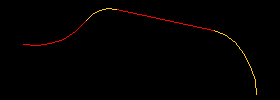FIGURE 1 An approximate curve is a spline which closely approximates other curves or edges to form a new curvature continuous representation.   Figure 1 shows a curvature discontinuous curve. The segments are tangent, but they are not curvature continuous. Figure 2 shows an approximate curve created over the top (referencing) the curve of Figure 1.  It is the cyan (light blue) one.  You can see (sort of) the coincident colors.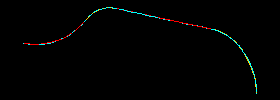FIGURE 2 Notes: By Pro/E definition, an approximate curve is coincident at the segment end points, but may vary from the rest of the curve to attain curvature continuity.  In layman’s terms that means there is not a step function in the curvature. For illustrative purposes, the curve segments shown here are different colors.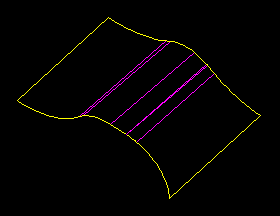FIGURE 3 Why is this useful? In the example of Figure 3, the quilt was created using 2 sketched curves.   This results in several surface "patches" as can be seen.  The quilt of Figure 4 is essentially the same except that it was created using curvature continuous curves.  Notice that this quilt does not have the surface patches. Surfaces patches such as these are not intrinsically bad, however, when they are small like those of Figure 3, they can cause problems with future references, later geometry, and create headaches with FEA and CAM.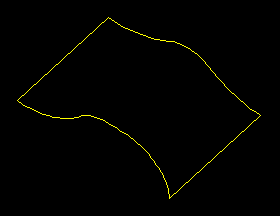FIGURE 4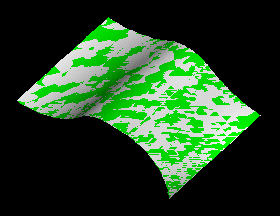FIGURE 5 In case you are skeptical about these surfaces, Figure 5 shows the 2 quilts on top of one another. Can you tell which is which? Actually, the curvature continuous quilt is green, and in Pro/E language, the oscillating colors as seen here are it's way of showing that the surfaces are extremely close to coincident. This example was done with surfaces, though the same techniques are also valid with solids.   Please see the November 1998 Tip for another example.   So how do you create curvature continuous curves?  There are 2 ways: Using datum curves or existing geometry edges. Create a datum curve or set of tangent curves. Use  FEATURE / CREATE / DATUM / CURVE / COMPOSITE / DONE / APPROXIMATE  then pick the required curves or geometry edges. For use in sketcher.  (Especially useful when working with solids. In sketcher, sketch the exact geometry desired. Make sure it regenerates successfully. Use  SKETCH / ADV GEOMETRY / SPLINE / APPROX CHAIN  then select the sketched entities you wish to be included in the approximate chain.  Note:  The sketch may not look different, but it is now a spline driven by the dimensions of the original exact geometry. Regenerate (if necessary) and continue with the feature creation. Cautions and other thoughts about using approximate curves: Don't mix exact geometry with approximate geometry.  In other words, if you use an approximate curve to construct geometry, don't create additional geometry in the same area using the exact version of the approximate curve. Don't make approximate curves that reference straight lines unless the straight portion is small with respect to the curved sections. Don't use non-tangent curves to create approximations.  This will only cause trouble down the road. Always look at the curve to determine if the result will work as desired.   Use  INFO / CURVE ANALYSIS  for a graphic demonstration of curvature. Examine the nature of the geometry you are trying to create.  Sometimes exact curves are the best solution, sometimes approximate, and sometimes an explicit spline or conic is best. Good luck using these approximate techniques.

.

SYNTHESIS ENGINEERING SERVICES INC. : (719) 380-1122